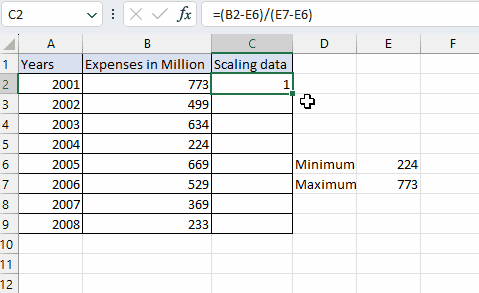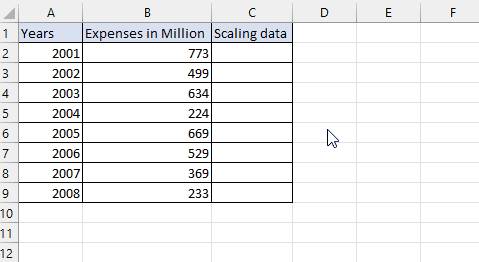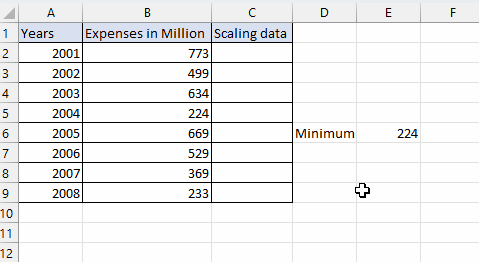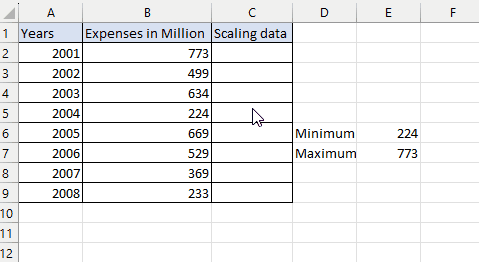# How to scale data in Excel

In this tutorial, we are using a dataset of the yearly expenses of a factory in millions to show how to scale data.Data scaling in Excel refers to the process of adjusting the range or scale of data to ensure comparability. It is necessary to prevent variables with larger magnitudes from dominating analyses or models. By scaling the data, variables are transformed to a common scale, allowing fair comparisons and avoiding bias. Scaling methods in Excel include Min-Max Scaling, Z-score Standardization, and more. Data scaling is crucial for accurate analysis, modeling, and visualization in Excel.

### Step 1 – Write the data’s minimum value– To write the minimum value write the minimum in a separate cell outside the dataset
– In front of that cell enter the formula =MIN(range)  in the selected cell.
– Replace “range” with the actual data range by dragging the selection from the start to the end of the data.
– Press Enter to calculate and display the minimum value.

### Step 2– Write the data’s maximum value– To write the maximum value, write the maximum in a separate cell outside the dataset
– In front of that cell enter the formula Enter the formula “=MAX(range)” in the selected cell.
– Replace “range” with the actual data range by dragging the selection from the start to the end of the data.
– Press Enter to calculate and display the maximum value.

### Step 3 – Scale data– To scale  the data fill the values in the formula =(X-Xmin) / (Xmax-Xmin)
– Here X represents the value to be scaled
– Xmin is the minimum or the lowest value
– Xmax is the maximum or the highest value
– Press Enter after inputting the values.

### Step 4 – Apply the function to all cells in the column– In order to carry out the function throughout the column lock the values.
– Select Xmax and Xmin in the formula.
– Press F4.
– This will lock the values.
– Move your cursor to the bottom right corner of the cell which contains the scaled value until it turns into a small black “+” sign.
– Click and drag the cursor down or across the range of cells where you want to apply the formula.